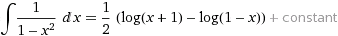# Basic differential equation question

## Homework Statement

Solve the equation:

y' = (1-y2)/ (1-x2) , y(0) = 3

## Homework Equations, log(x) is natural logarithm

## The Attempt at a Solution

After solving, my final result was 3 -y+x-3xy =0, but the given answer is 8x2+y2=9.. can someone please kindly check the answer for me? I checked it several times but I still couldn't find anything wrong with my solution..

## Answers and Replies

Have you checked to see whether your solution satisfies the initial condition or the original ODE?

I tried it too and I got your answer. Then I checked Wolfram Alpha and it confirmed it.

epenguin
Homework Helper
Gold Member
Me too and confirm check if the 'given answer' satisfies the d.e.(Useful habit too).